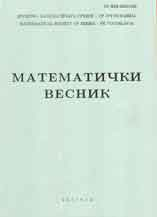eLibrary of Mathematical Instituteof the Serbian Academy of Sciences and Arts> Home / All Journals / Journal /Matematički VesnikPublisher: Društvo matematičara Srbije, BeogradISSN: 0025-5165 (Print), 2406-0682 (Online)Issue: 74_2Date: 2022Journal Homepage

 On singular fifth-order boundary value problems with deficiency indices $\boldsymbol{(5,5)}$ 79 - 88 E. Uğurlu and K. Taş
AbstractKeywords: fifth-order equation; eigenvalue problems; boundary conditions.MSC: 34B05 34B40; 34B09
 Iterative method for finding zeros of monotone mappings and fixed point of certain nonlinear mapping 89 - 100 J.N. Ezeora, C. Izuchukwu and R.C. Ogbonna
AbstractKeywords: monotone operators; strict-pseudo contractive mappings; strict-pseudo nonspreading mappings; resolvents; Hilbert space.MSC: 47H04 47H06; 47H15; 47H17; 47J25
 Approximation of generalized PǍltǎnea and Heilmann-type operators 101 - 109 S. Kumar and N. Deo
AbstractKeywords: difference operators; generalized Pǎltǎnea type operators; Heilmann type operators; modulus of continuity; rate of convergence.MSC: 41A25 26A15;41A30
AbstractKeywords: $\mathcal I^{*}\text{-}\alpha$ convergence; $\mathcal I^*$-exhaustiveness; sequence of metric functions; ideal convergence.MSC: 40A35 26A03
 Bounds for the $\boldsymbol{A_{\alpha}}$-spectral radius of a digraph 119 - 129 H. A. Ganie and M. Baghipur
 Fixed points of almost Suzuki type $\boldsymbol{\mathcal{Z}_s}$-contractions in $S$-metric spaces 130 - 140 G. V. R. Babu, P. Durga Sailaja and G. Srichandana
AbstractKeywords: $S$-metric space; $\mathcal{Z}$-contraction; simulation function; $\mathcal{Z}_s$-contraction; almost Suzuki type $\mathcal{Z}_s$-contraction.MSC: 47H10 54H25